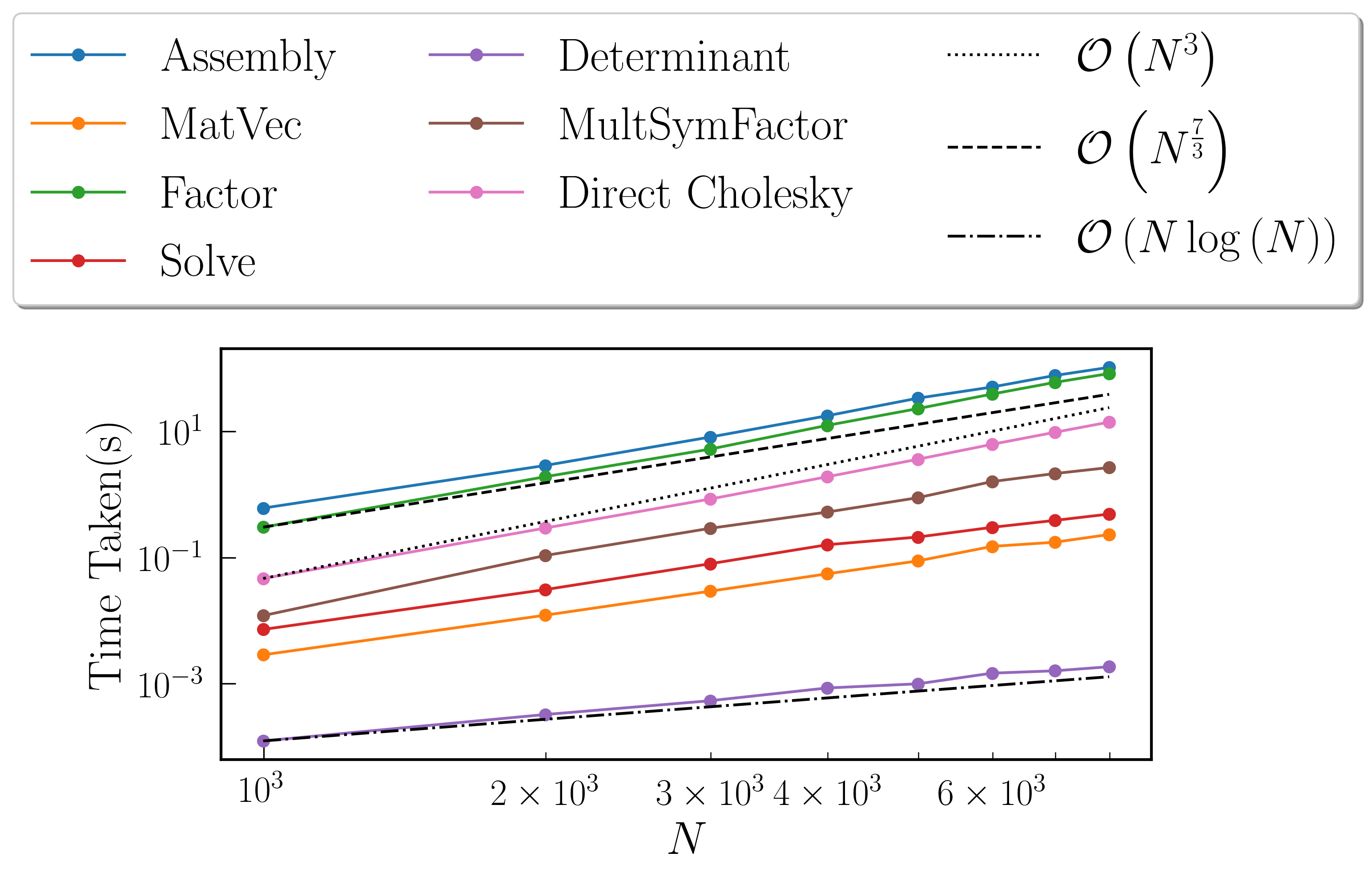# Benchmarks¶

All the following benchmarks have been carried out on an i7-8750H(with OpenMP enabled, this is 12 threads), with Intel’s icpc (ICC) 19.0.1.144 compiler and Eigen version 3.3.7, with DTYPE set to double. The compiler flags that were utilized are the same are those mentioned in the CMakeLists.txt file.

Presented below are the results as obtained when using different kernels:

## Gaussian Kernel¶

The Gaussian Kernel is given by $$K(i, j) = \sigma^2 \delta_{ij}^2 + \exp(-||x_i - x_j||^2)$$. For these benchmarks, we take $$\sigma = 10$$ with $$x$$ being set as a sorted random vector $$\in (-1, 1)$$. Using the plotTree function of this library, we can look at the rank structure for this matrix. The following diagram is obtained with $$N = 10000$$, $$M = 500$$ and tolerance $$10^{-12}$$The green blocks are low-rank blocks. Their intensity of colour shows their degree of “low-rankness”. Additionally, the rank has been displayed in each of these blocks. The red blocks are full-rank blocks and would have the rank of $$M = 500$$

### Time Taken vs Tolerance¶

These benchmarks were performed for size of the matrix $$N = 1000000$$, with the size of the leaf node set to $$M = 100$$.

#### Fast Factorization¶

Tolerance Assembly(s) MatVec(s) Factorize(s) Solve(s) Determinant(s)
$$10^{-2}$$ 1.65059 11.4442 1.47112 0.321805 0.0300901
$$10^{-4}$$ 1.82825 8.33693 1.94887 0.337377 0.03039
$$10^{-6}$$ 1.92681 12.4077 2.33157 0.346198 0.0300648
$$10^{-8}$$ 2.09475 11.5901 2.74718 0.361579 0.0338411
$$10^{-10}$$ 2.28711 11.8123 3.22611 0.375279 0.0296249
$$10^{-12}$$ 2.54764 11.2157 3.89779 0.398319 0.0305111
$$10^{-14}$$ 2.95124 8.55489 5.01199 0.431082 0.0309851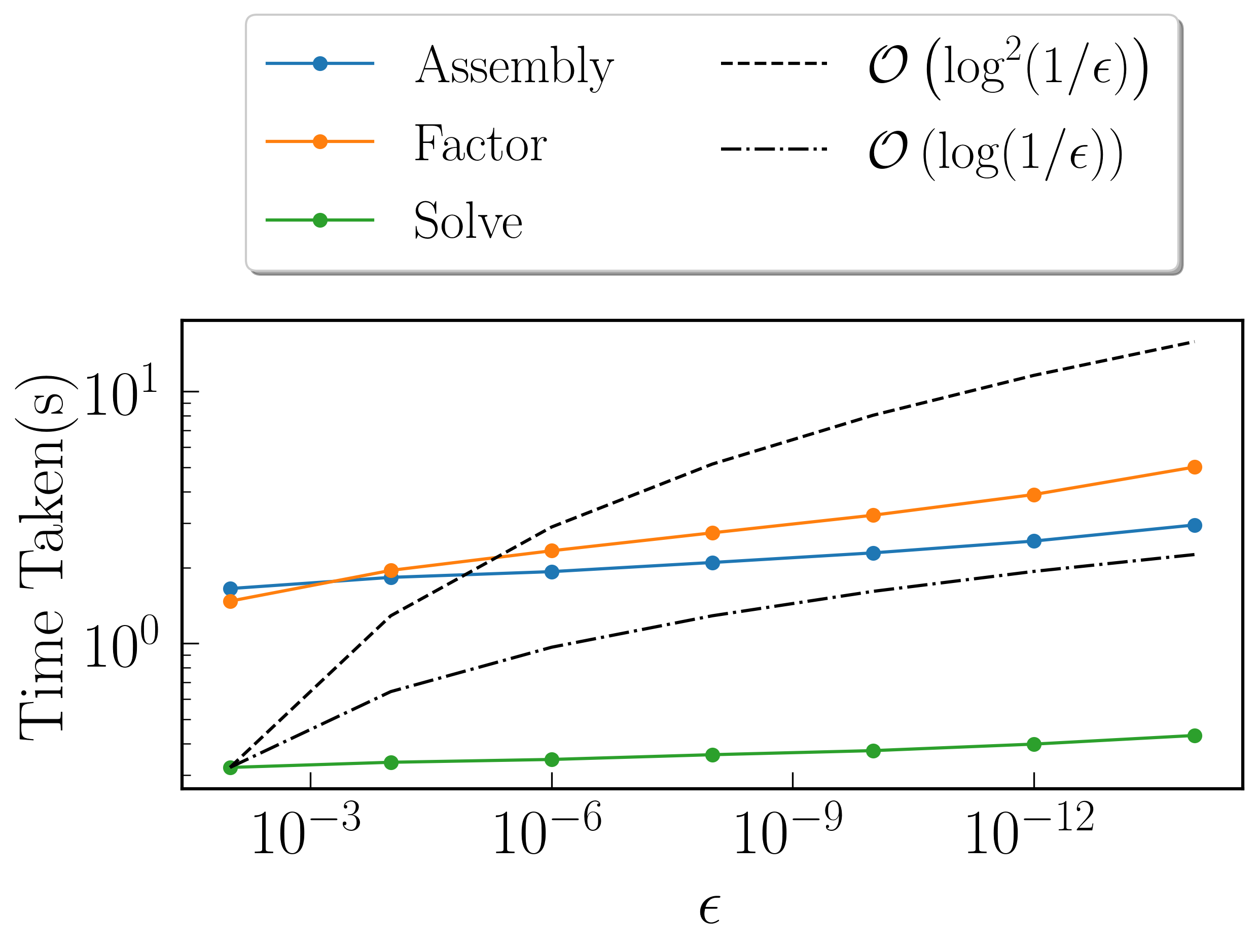#### Fast Symmetric Factorization¶

Tolerance Assembly(s) MatVec(s) Factorize(s) Solve(s) Determinant(s) MultSymFactor(s)
$$10^{-2}$$ 1.61076 11.4741 1.17726 0.387992 0.0366111 0.226509
$$10^{-4}$$ 1.81511 8.08747 1.56692 0.399085 0.0328679 0.249969
$$10^{-6}$$ 1.91956 12.3341 1.83361 0.418334 0.031215 0.266352
$$10^{-8}$$ 2.07343 11.2653 2.29376 0.440591 0.0327439 0.288697
$$10^{-10}$$ 2.24097 11.7877 2.86431 0.464687 0.0305729 0.339399
$$10^{-12}$$ 2.57603 11.3027 3.66516 0.494536 0.031522 0.393104
$$10^{-14}$$ 2.90611 7.89484 4.93738 0.537149 0.030225 0.393285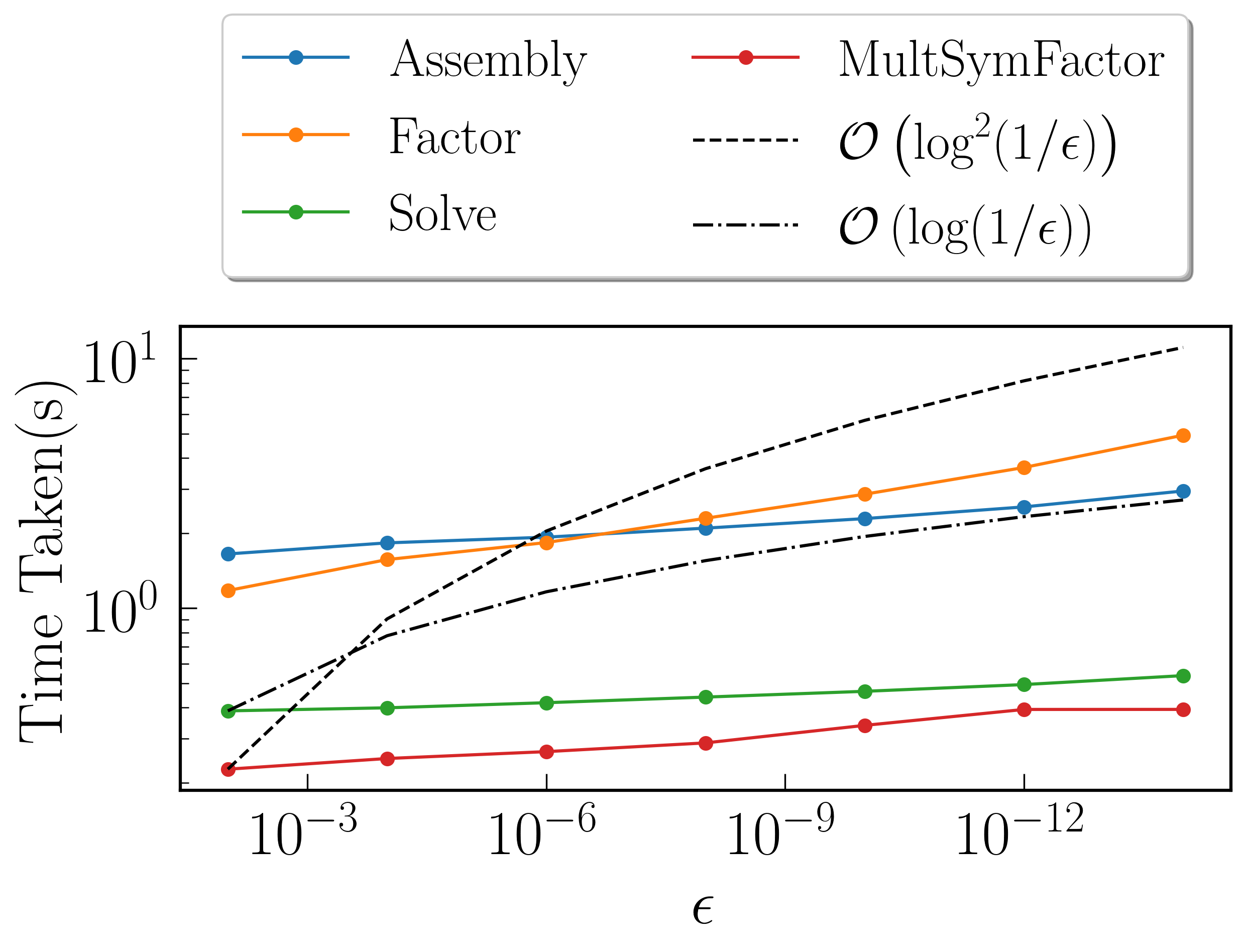### Time Taken vs Size of Matrix¶

For these benchmarks, the leaf size was fixed at $$M = 100$$, with tolerance set to $$10^{-12}$$

#### Fast Factorization¶

$$N$$ Assembly(s) MatVec(s) Factorize(s) Solve(s) Determinant(s) Direct LU(s)
$$10^{3}$$ 0.00345016 0.000463963 0.00121403 0.000246048 2.09808e-05 0.024302
$$5 \times 10^{3}$$ 0.00954294 0.000818014 0.00755906 0.00179601 0.000159979 1.61282
$$10^{4}$$ 0.0180159 0.00202203 0.103507 0.003834 0.000344992 10.4102
$$5 \times 10^{4}$$ 0.109816 0.0147851 0.103266 0.022316 0.00227404 N/A
$$10^{5}$$ 0.202525 0.066885 0.239639 0.0450559 0.00451112 N/A
$$5 \times 10^{5}$$ 1.19365 3.68382 1.6615 0.206754 0.015748 N/A
$$10^{6}$$ 2.53519 11.1435 3.93549 0.399695 0.0303771 N/A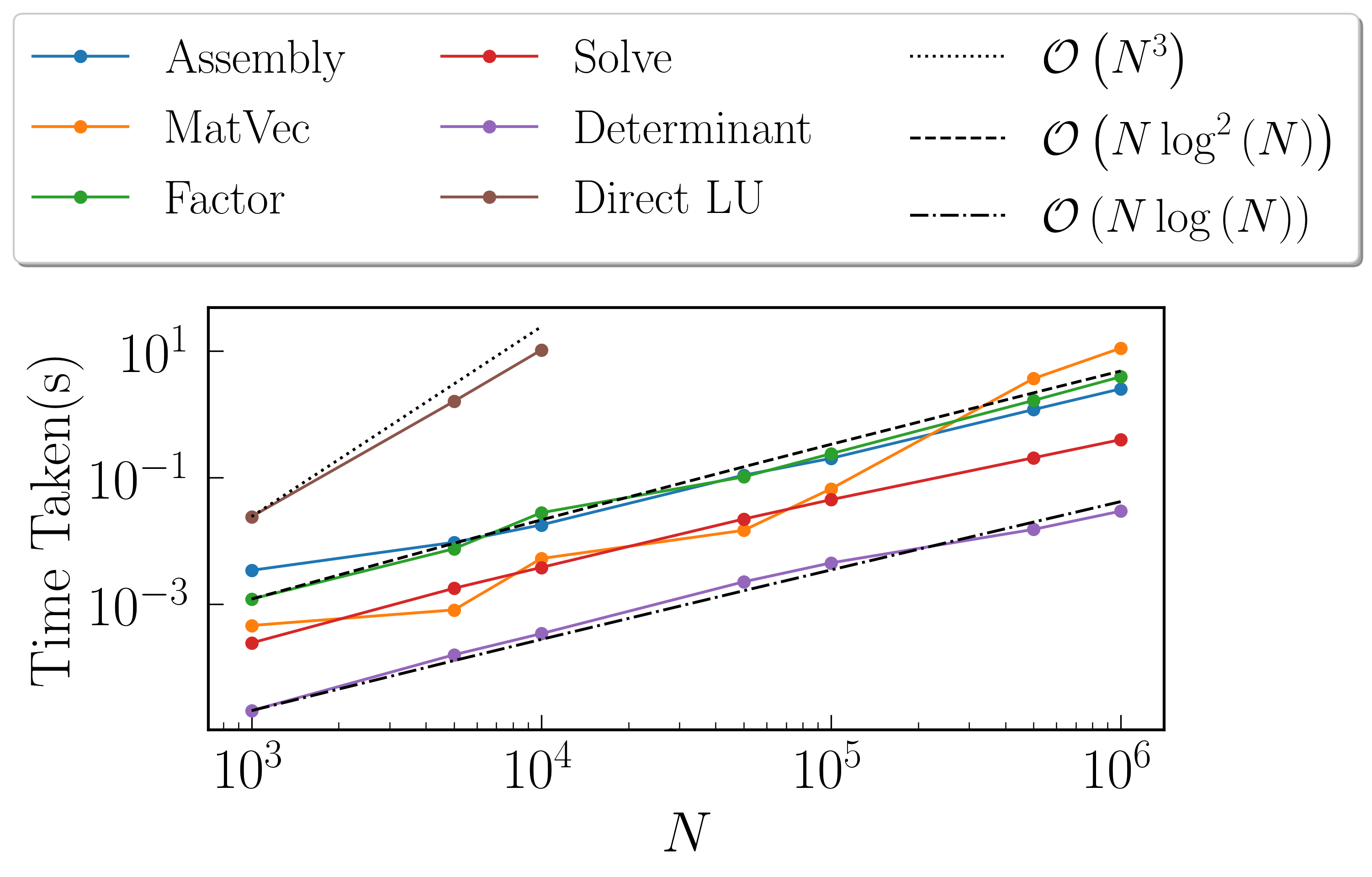#### Fast Symmetric Factorization¶

$$N$$ Assembly(s) MatVec(s) Factorize(s) Solve(s) Determinant(s) MultSymFactor(s) Direct Cholesky(s)
$$10^{3}$$ 0.00344396 0.000510931 0.00103807 0.00030303 2.19345e-05 0.000180006 0.0316679
$$5 \times 10^{3}$$ 0.00925708 0.000812054 0.00626493 0.00209403 0.000108004 0.00113392 2.35399
$$10^{4}$$ 0.0183232 0.00199389 0.010865 0.00471711 0.000352859 0.00263691 18.5745
$$5 \times 10^{4}$$ 0.0946209 0.0151899 0.0787759 0.0285201 0.00230503 0.0157571 N/A
$$10^{5}$$ 0.203769 0.0659761 0.183974 0.058074 0.00438595 0.03263 N/A
$$5 \times 10^{5}$$ 1.18639 3.67825 1.47418 0.245743 0.0180571 0.162066 N/A
$$10^{6}$$ 2.53567 11.2973 3.56786 0.488049 0.0311899 0.377352 N/A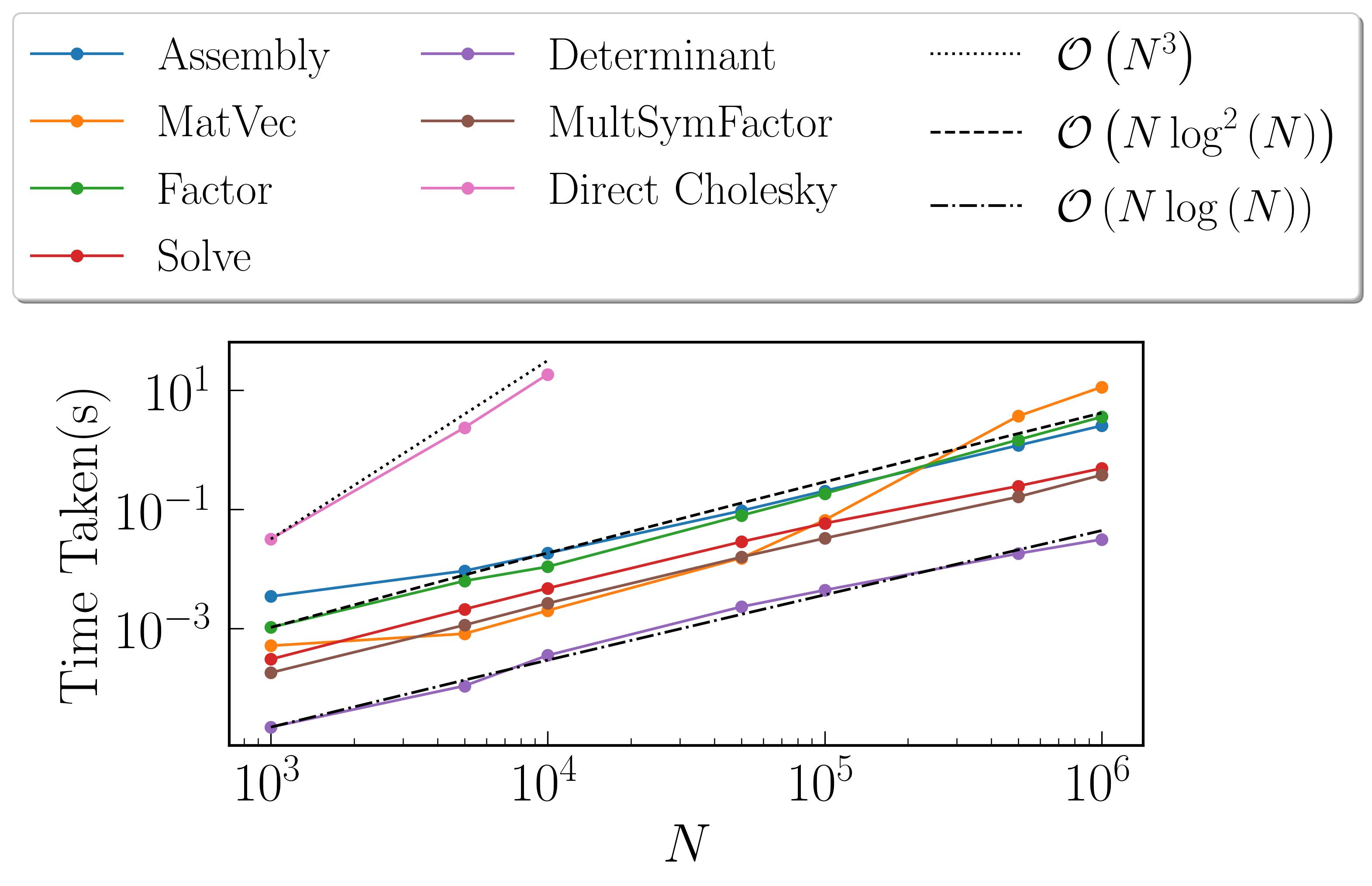## Matérn Kernel¶

Kernel considered is given by $$K(r) = \sigma^2 \left(1 + \frac{r \sqrt{5}}{\rho} + \frac{5 r^2}{3 \rho^2}\right)\exp{\left(-\frac{r \sqrt{5}}{\rho}\right)}$$. For these benchmarks, we take $$\sigma = 10$$, $$\rho = 5$$, where $$r = ||x_i - x_j||$$ with $$x$$ being set as a sorted random vector $$\in (-1, 1)$$. Using plotTree for $$N = 10000$$, $$M = 500$$ and tolerance $$10^{-12}$$, we see this rank structure### Time Taken vs Tolerance¶

These benchmarks were performed for size of the matrix $$N = 1000000$$, with the size of the leaf node set to $$M = 100$$.

#### Fast Factorization¶

Tolerance Assembly(s) MatVec(s) Factorize(s) Solve(s) Determinant(s)
$$10^{-2}$$ 1.70237 13.8247 1.3231 0.388983 0.042177
$$10^{-4}$$ 1.93746 14.0274 1.37327 0.401342 0.0430369
$$10^{-6}$$ 1.99264 9.29146 1.6509 0.413971 0.0420959
$$10^{-8}$$ 2.04502 13.6249 1.80135 0.417019 0.043962
$$10^{-10}$$ 2.08538 14.7541 2.1616 0.455189 0.0420899
$$10^{-12}$$ 2.28954 9.11655 2.27049 0.431815 0.043808
$$10^{-14}$$ 2.19898 13.821 2.74798 0.466761 0.0431418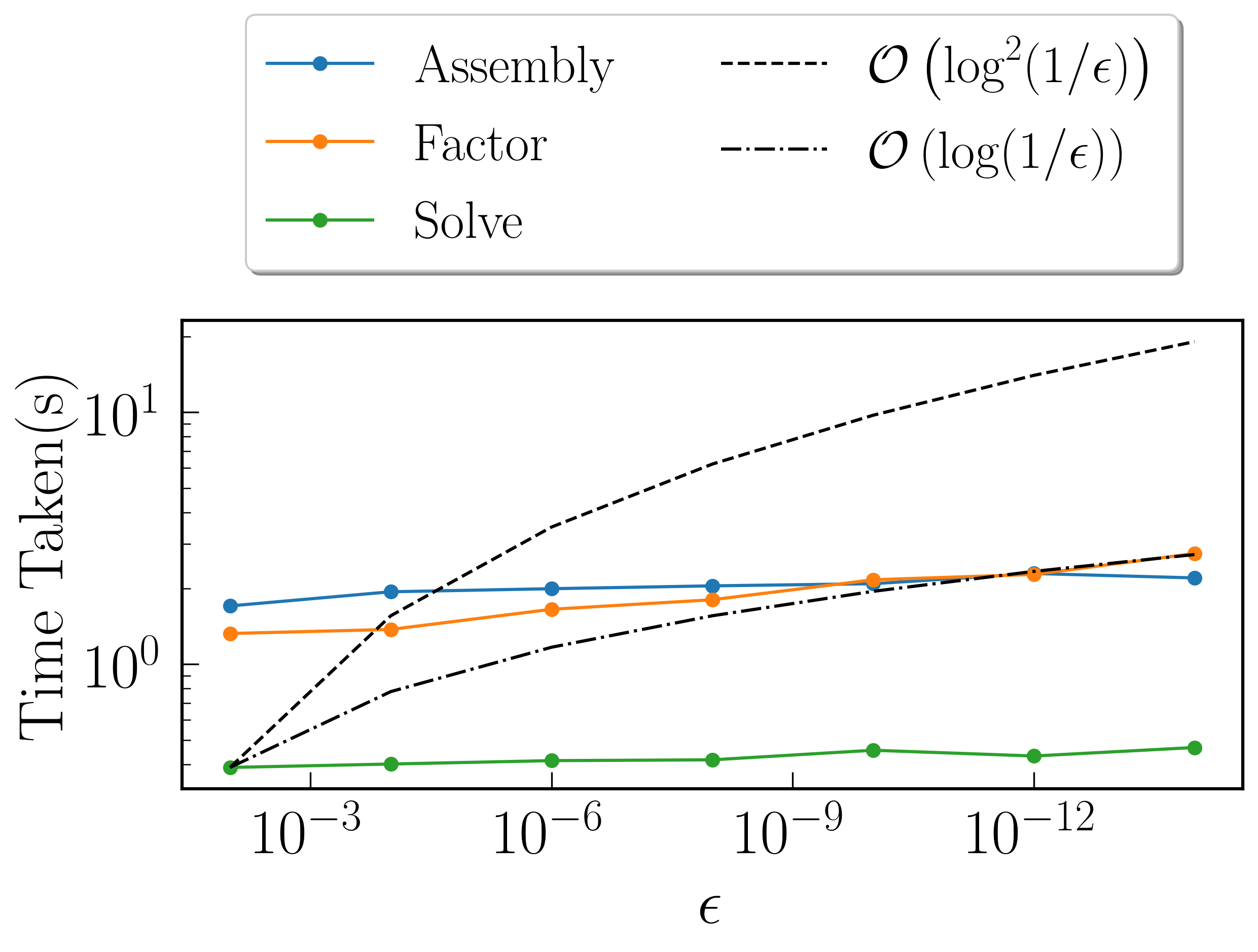#### Fast Symmetric Factorization¶

Tolerance Assembly(s) MatVec(s) Factorize(s) Solve(s) Determinant(s) MultSymFactor(s)
$$10^{-2}$$ 1.65146 13.4722 0.722689 0.461396 0.0417881 0.257583
$$10^{-4}$$ 1.87788 13.6014 0.778202 0.471056 0.041806 0.263908
$$10^{-6}$$ 1.93905 8.81335 0.836078 0.478072 0.0427818 0.268437
$$10^{-8}$$ 2.05821 13.4592 1.05975 0.496589 0.0437939 0.294927
$$10^{-10}$$ 2.0032 14.3409 1.31922 0.507549 0.0424139 0.296023
$$10^{-12}$$ 2.23442 8.84984 1.51609 0.533495 0.0427949 0.311331
$$10^{-14}$$ 2.18632 13.6219 1.95092 0.551657 0.0439069 0.342182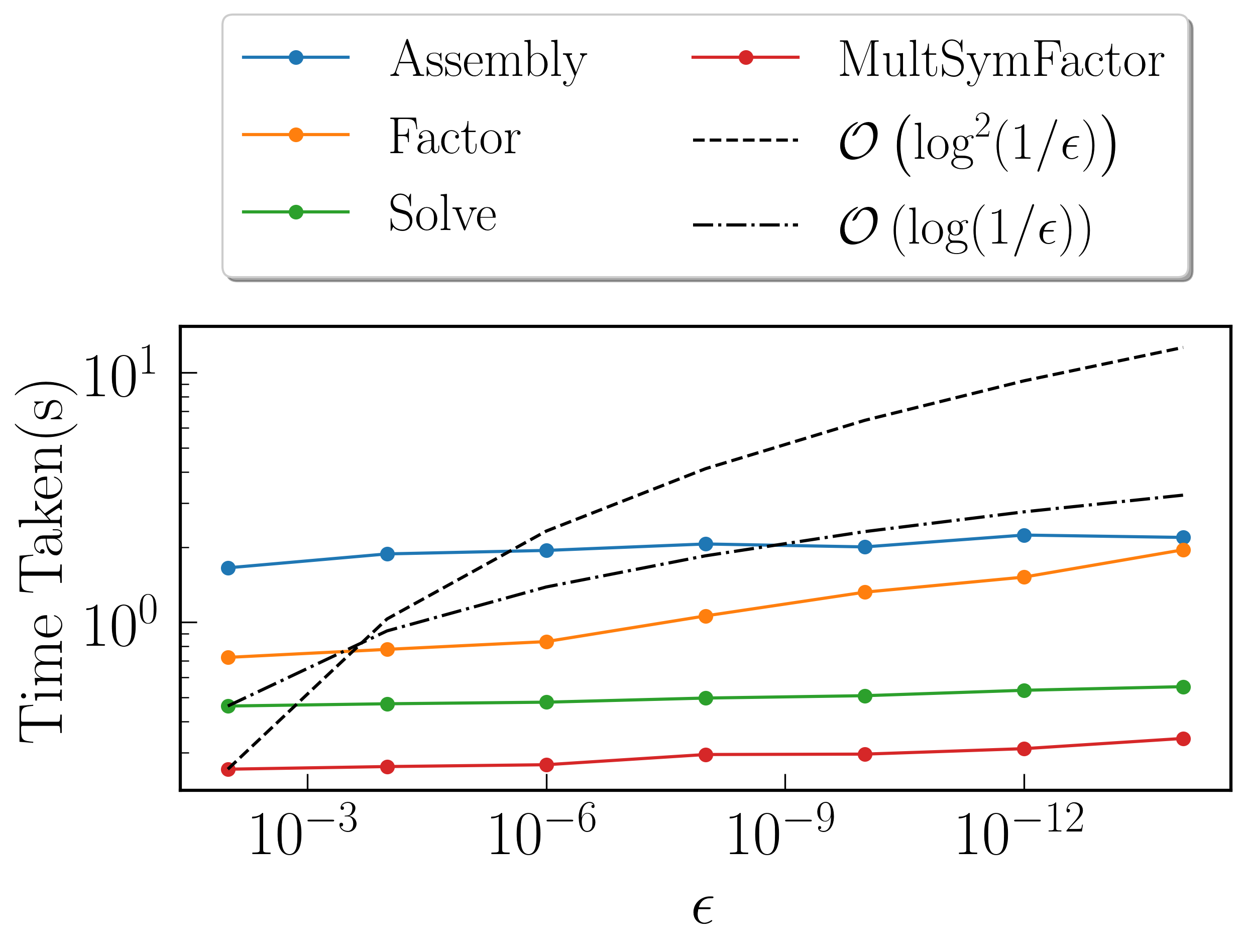### Time Taken vs Size of Matrix¶

For these benchmarks, the leaf size was fixed at $$M = 100$$, with tolerance set to $$10^{-12}$$

#### Fast Factorization¶

$$N$$ Assembly(s) MatVec(s) Factorize(s) Solve(s) Determinant(s) Direct LU(s)
$$10^{3}$$ 0.00927687 0.0001921 0.0011642 0.000297 3.19481e-05 0.0489709
$$5 \times 10^{3}$$ 0.0159199 0.0007879 0.00726509 0.002069 0.000204086 2.52755
$$10^{4}$$ 0.026196 0.0020630 0.0235729 0.005370 0.000522852 16.0086
$$5 \times 10^{4}$$ 0.098814 0.0144801 0.106045 0.027053 0.00375605
$$10^{5}$$ 0.180091 0.0756569 0.19264 0.054170 0.00687695
$$5 \times 10^{5}$$ 1.10963 3.33762 0.943877 0.234129 0.0219009
$$10^{6}$$ 2.25833 9.01339 2.33021 0.450053 0.041976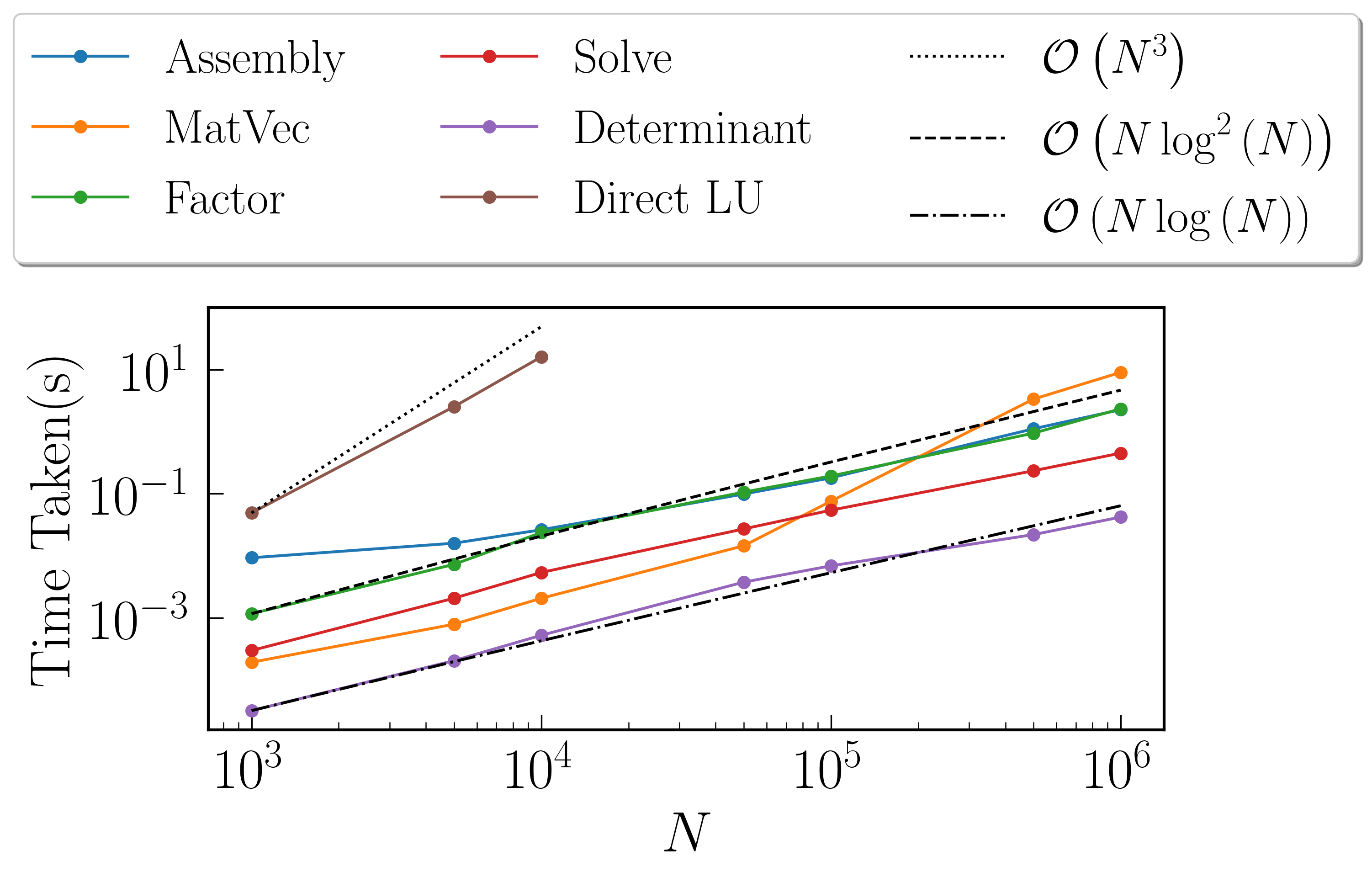#### Fast Symmetric Factorization¶

$$N$$ Assembly(s) MatVec(s) Factorize(s) Solve(s) Determinant(s) MultSymFactor(s) Direct Cholesky(s)
$$10^{3}$$ 0.0066328 0.000208855 0.000833988 0.00034499 2.81334e-05 0.000160933 0.0281229
$$5 \times 10^{3}$$ 0.0103149 0.000798941 0.00359011 0.00228715 0.000156879 0.00105405 0.231569
$$10^{4}$$ 0.02724 0.00200987 0.0175741 0.00552893 0.000396013 0.00261402 1.05882
$$5 \times 10^{4}$$ 0.08972 0.0151231 0.044107 0.034517 0.00314713 0.0162551 N/A
$$10^{5}$$ 0.192696 0.067266 0.0933969 0.0709021 0.0061872 0.0332701 N/A
$$5 \times 10^{5}$$ 1.09055 3.2381 0.612783 0.263855 0.024405 0.151778 N/A
$$10^{6}$$ 2.19711 8.79683 1.47177 0.545244 0.0434139 0.310443 N/A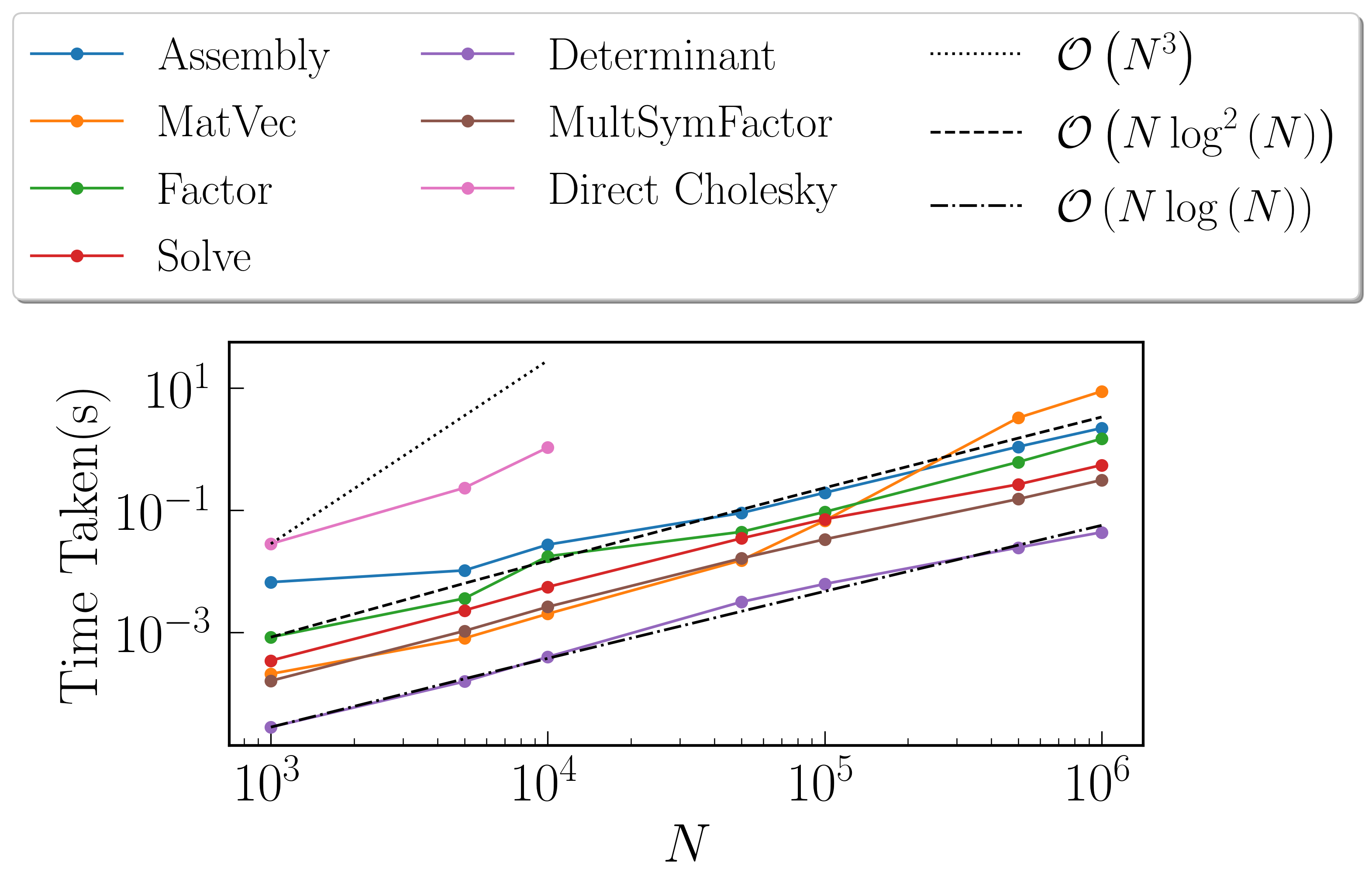## RPY Tensor¶

The RPY Tensor is given by

$\begin{split}K(i, j) = \begin{cases} \frac{k_B T}{6 \pi \eta a} \left[\left(1 - \frac{9}{32} \frac{r}{a}\right)\textbf{I} + \frac{3}{32a} \frac{\textbf{r} \otimes \textbf{r}}{r}\right] , & \text{if } r < 2a \\ \frac{k_B T}{8 \pi \eta r} \left[\textbf{I} + \frac{\textbf{r} \otimes \textbf{r}}{r^2} + \frac{2a^2}{3r^2}\left(\textbf{I} - 3 \frac{\textbf{r} \otimes \textbf{r}}{r^2}\right)\right] , & \text{if } r \geq 2a \\ \end{cases}\end{split}$

where $$r = ||\textbf{r}_i - \textbf{r}_j||$$ with $$\textbf{r}$$ being set as a sorted random matrix $$\in (-1, 1)$$ with the number of columns set equal to the dimension considered. For these benchmarks, we take $$k_B = T = \eta = 1$$. For $$a$$, we find the minimum of the interaction distances between all particles $$r_{min}$$ and set $$a = \frac{r_{min}}{2}$$. This means that for the considered case, the RPY tensor simplifies to:

$\begin{split}K(i, j) = \begin{cases} \frac{k_B T}{6 \pi \eta a} \textbf{I} , & \text{if } i = j \\ \frac{k_B T}{8 \pi \eta r} \left[\textbf{I} + \frac{\textbf{r} \otimes \textbf{r}}{r^2} + \frac{2a^2}{3r^2}\left(\textbf{I} - 3 \frac{\textbf{r} \otimes \textbf{r}}{r^2}\right)\right] , & \text{if } i \neq j \\ \end{cases}\end{split}$

We have used plotTree to reveal the rank structure for the problems below when considering matrix size $$N = 10000$$, leaf size $$M = 500$$ and tolerance $$10^{-12}$$.

### Time Taken vs Size of Matrix¶

For these benchmarks, the leaf size was fixed at $$M = 100$$, with tolerance set to $$10^{-12}$$

#### Fast Factorization¶

$$N$$ Assembly(s) MatVec(s) Factorize(s) Solve(s) Determinant(s) Direct LU(s)
$$10^{3}$$ 0.0284998 0.0002059 0.00317788 0.000329 2.19345e-05 0.022975
$$5 \times 10^{3}$$ 0.124997 0.0019462 0.028585 0.003378 0.000226021 1.57937
$$10^{4}$$ 0.284125 0.0044059 0.0781479 0.007328 0.000458002 11.3985
$$5 \times 10^{4}$$ 1.60538 0.033412 0.67361 0.047978 0.001616
$$10^{5}$$ 5.49457 0.145549 2.47014 0.254623 0.00333095
$$5 \times 10^{5}$$ 28.6773 3.55899 17.4057 0.818555 0.0195651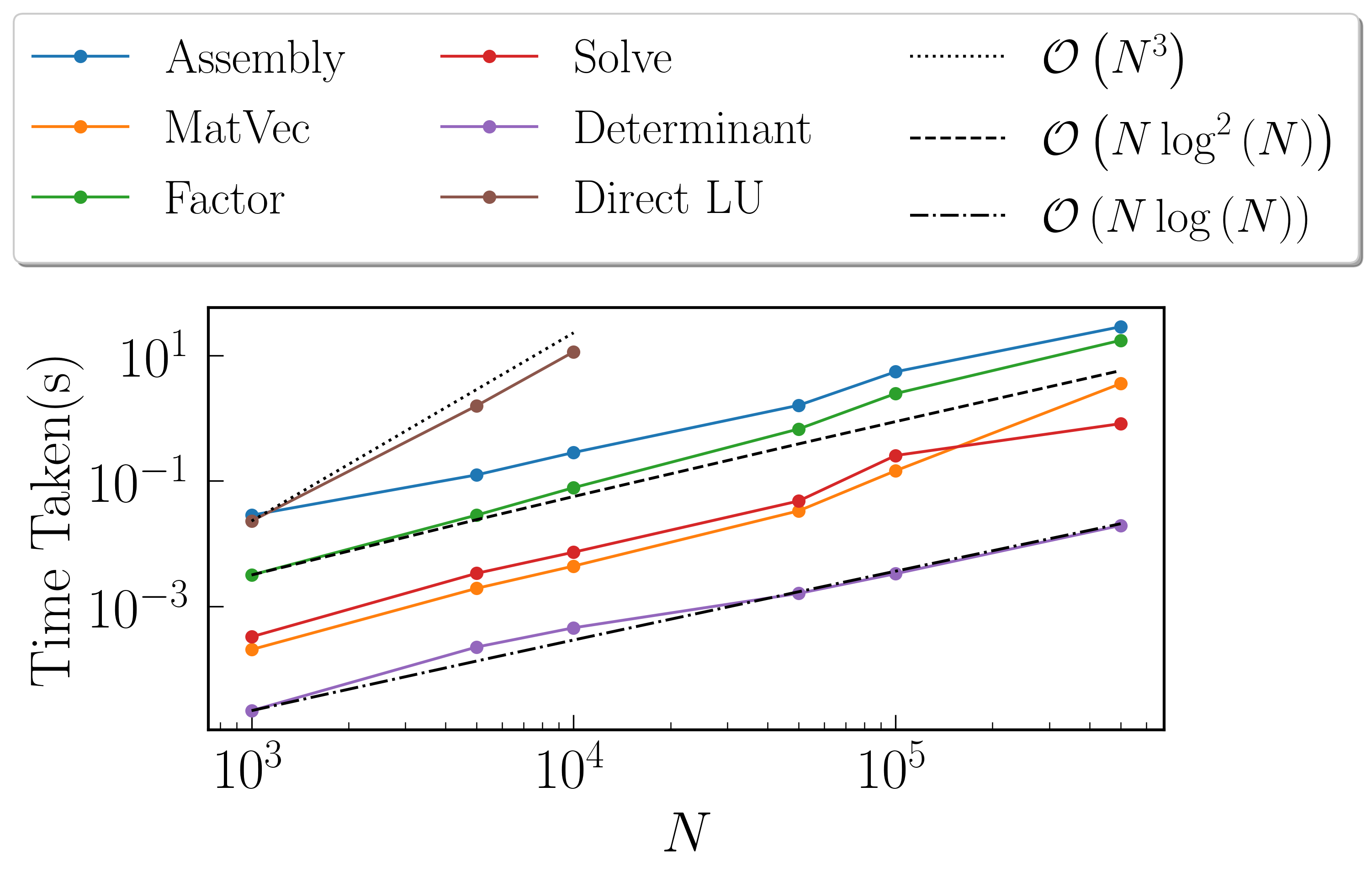#### Fast Symmetric Factorization¶

$$N$$ Assembly(s) MatVec(s) Factorize(s) Solve(s) Determinant(s) MultSymFactor(s) Direct Cholesky(s)
$$10^{3}$$ 0.0468209 0.00031209 0.008219 0.00095796 3.19481e-05 0.0005548 0.034517
$$5 \times 10^{3}$$ 0.216226 0.00294399 0.042495 0.00592899 0.000274181 0.0056932 3.34734
$$10^{4}$$ 0.47921 0.00559902 0.10963 0.0136352 0.00058794 0.0109739 12.3261
$$5 \times 10^{4}$$ 2.51609 0.0369091 0.609879 0.091403 0.00190592 0.069257 N/A
$$10^{5}$$ 5.30011 0.124498 1.83744 0.198894 0.00388098 0.161215 N/A
$$5 \times 10^{5}$$ 28.9266 3.54814 13.6953 1.03255 0.0130229 1.06126 N/A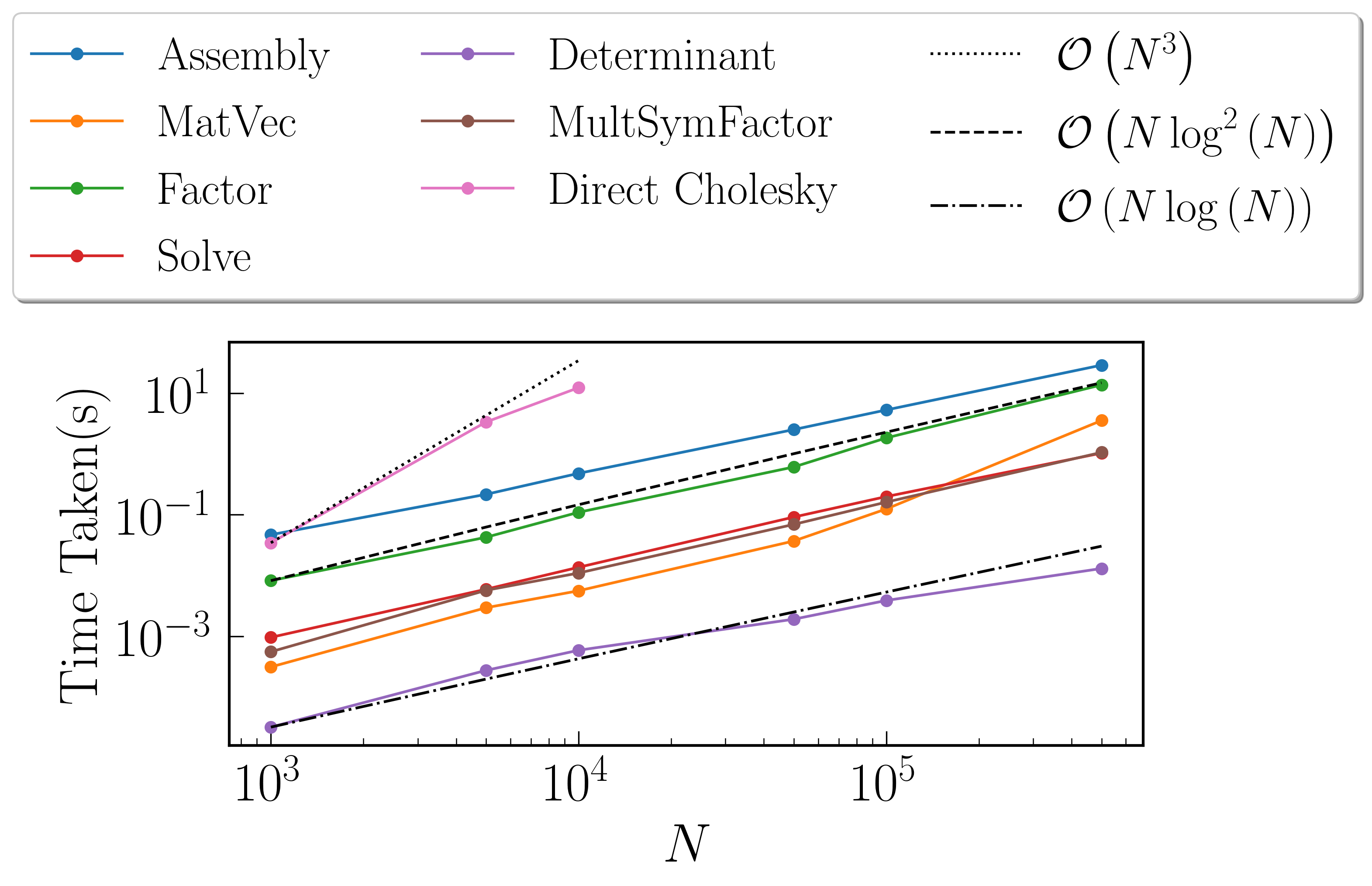### Time Taken vs Size of Matrix¶

For these benchmarks, the leaf size was fixed at $$M = 100$$, with tolerance set to $$10^{-12}$$

#### Fast Factorization¶

$$N$$ Assembly(s) MatVec(s) Factorize(s) Solve(s) Determinant(s) Direct LU(s)
$$10^{3}$$ 0.237684 0.0022819 0.161561 0.003957 0.000130177 0.0220509
$$2 \times 10^{3}$$ 0.854779 0.0138352 0.728164 0.013024 0.000378847 0.148836
$$4 \times 10^{3}$$ 3.31121 0.0200999 2.52401 0.037282 0.000866175 0.88069
$$8 \times 10^{3}$$ 15.0432 0.0863769 10.1511 0.120667 0.00184798 6.3137
$$1.6\times10^{4}$$ 63.8282 0.278127 53.9138 0.46551 0.0048461 55.4166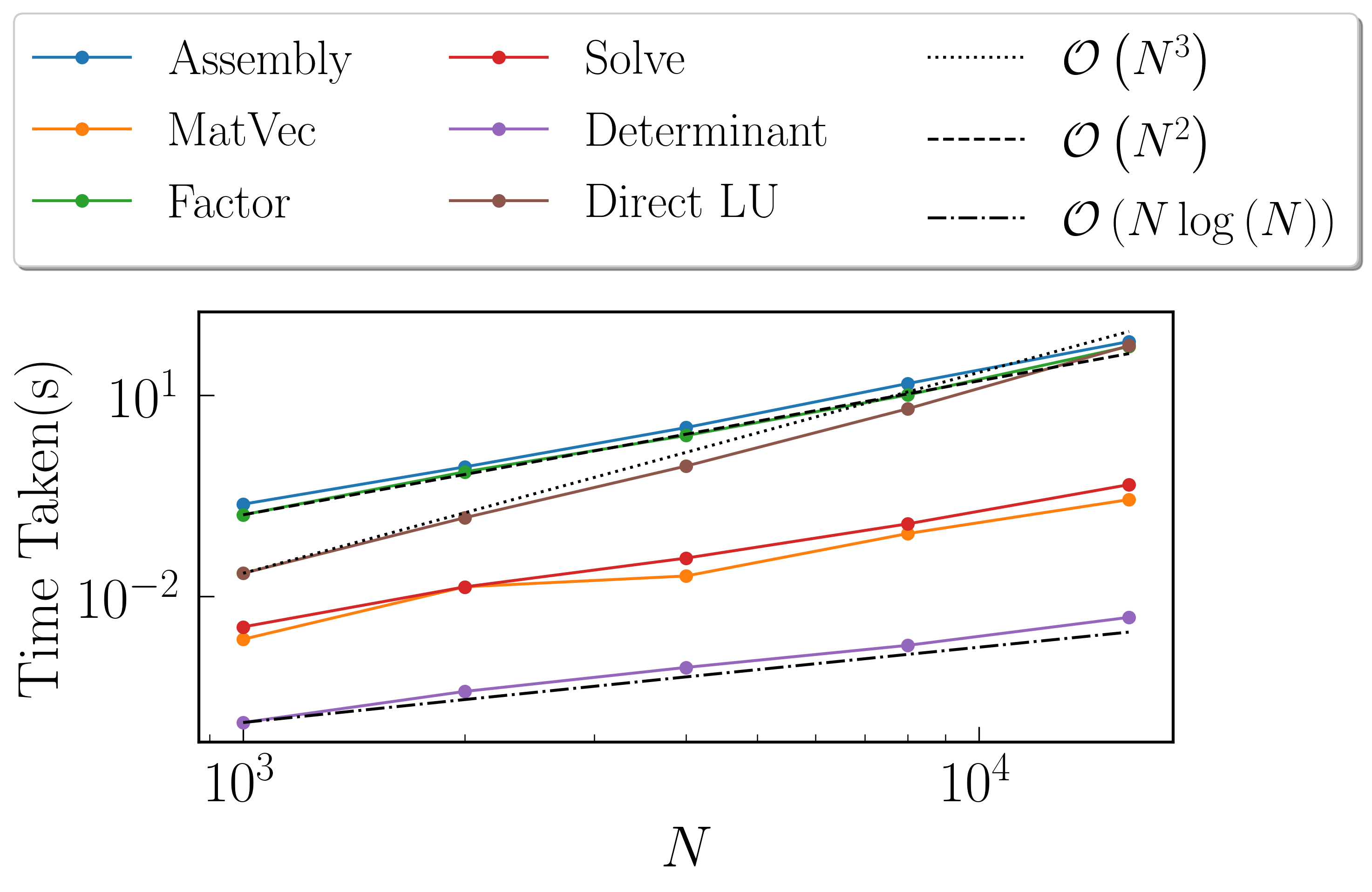#### Fast Symmetric Factorization¶

$$N$$ Assembly(s) MatVec(s) Factorize(s) Solve(s) Determinant(s) MultSymFactor(s) Direct Cholesky(s)
$$10^{3}$$ 0.375776 0.00342607 0.24704 0.00635099 0.000114918 0.0118291 0.026149
$$2 \times 10^{3}$$ 1.35015 0.00995803 1.05952 0.0212729 0.000280142 0.0441241 0.130154
$$4 \times 10^{3}$$ 4.89776 0.0418921 4.12168 0.107635 0.000642061 0.142907 1.49561
$$8 \times 10^{3}$$ 19.4326 0.0971079 16.3962 0.201411 0.00139117 0.547673 6.13806
$$1.6\times10^{4}$$ 79.2166 0.539779 66.3061 0.716309 0.00301003 2.18507 52.0411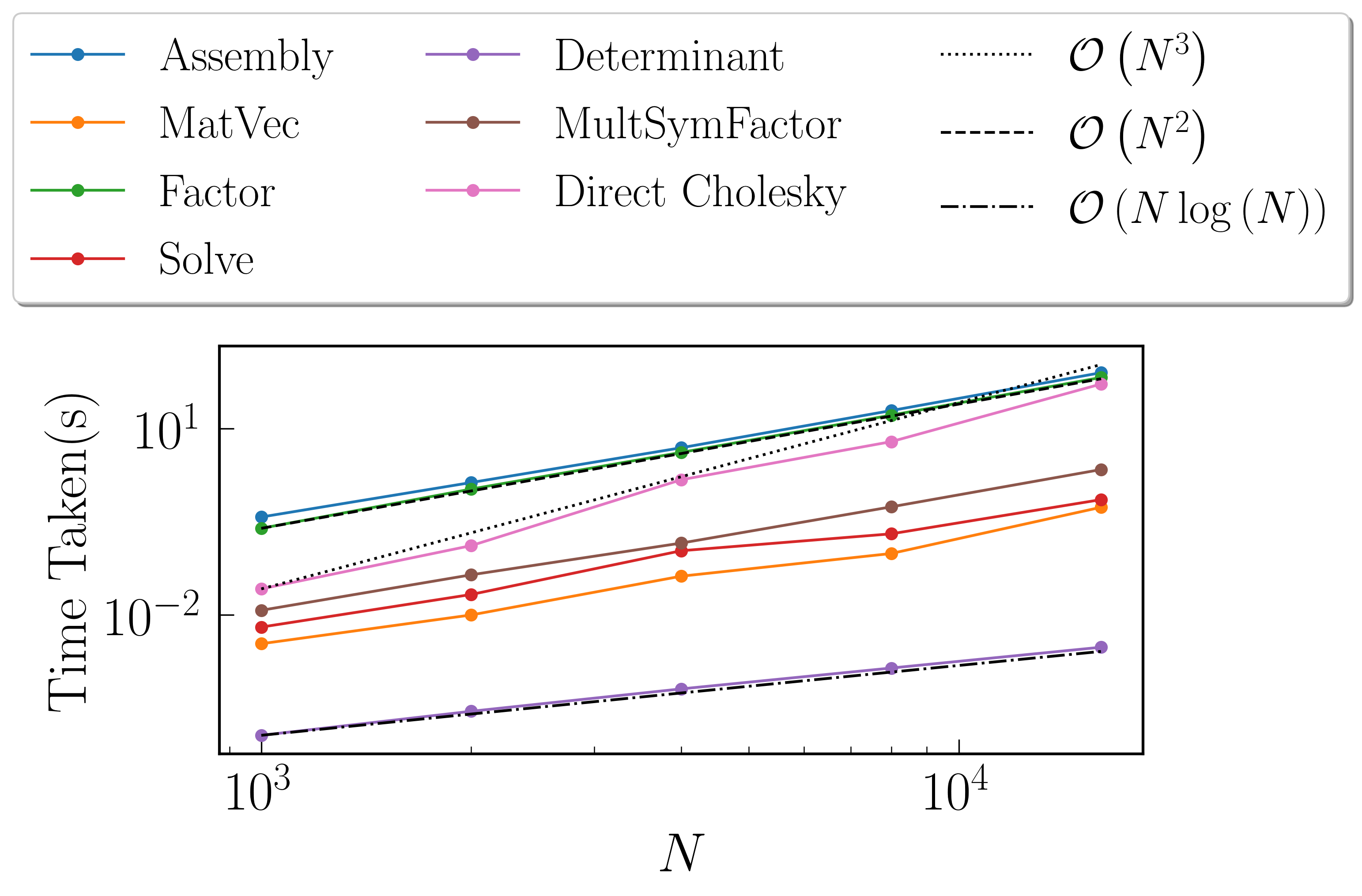### $$\texttt{dim} = 3$$¶For these benchmarks, the leaf size was fixed at $$M = 100$$, with tolerance set to $$10^{-12}$$

#### Fast Factorization¶

$$N$$ Assembly(s) MatVec(s) Factorize(s) Solve(s) Determinant(s) Direct LU(s)
$$999$$ 0.637549 0.0138719 0.357686 0.006175 0.000198841 0.0427179
$$2 \times 999$$ 2.67525 0.0112109 1.915 0.026588 0.000624895 0.209663
$$3 \times 999$$ 7.72688 0.0243111 5.49087 0.056867 0.000961065 0.608226
$$4 \times 999$$ 16.6299 0.0466208 12.8754 0.105617 0.00150394 1.26595
$$5 \times 999$$ 31.9858 0.078845 24.7647 0.169478 0.00192094 2.26981
$$6 \times 999$$ 48.5977 0.114865 41.1307 0.241243 0.00212693 3.69254
$$7 \times 999$$ 76.1404 0.159873 64.5563 0.32299 0.00276279 5.80262
$$8 \times 999$$ 105.803 0.238746 91.5038 0.405407 0.00317407 8.46848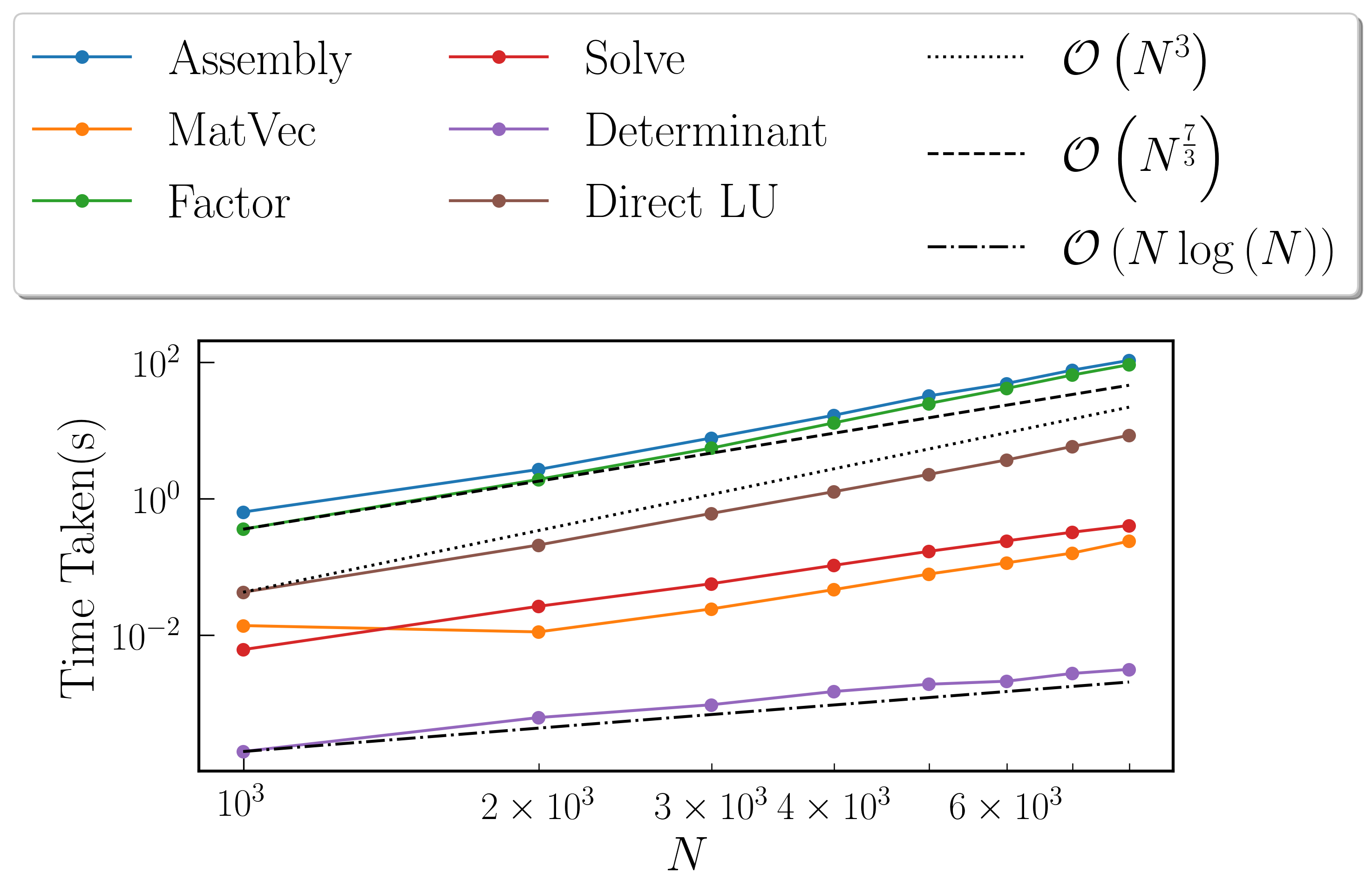#### Fast Symmetric Factorization¶

$$N$$ Assembly(s) MatVec(s) Factorize(s) Solve(s) Determinant(s) MultSymFactor(s) Direct Cholesky(s)
$$999$$ 0.60924 0.00287509 0.303544 0.00726318 0.000123978 0.012032 0.046663
$$2 \times 999$$ 2.88964 0.0122139 1.91556 0.0310731 0.000324965 0.107921 0.294039
$$3 \times 999$$ 8.15757 0.029355 5.2501 0.079915 0.000537872 0.291421 0.848032
$$4 \times 999$$ 17.7442 0.055275 12.4843 0.159855 0.000857115 0.527207 1.91446
$$5 \times 999$$ 33.741 0.08866 23.0541 0.211891 0.000997066 0.891842 3.62749
$$6 \times 999$$ 50.7127 0.150594 39.2222 0.302245 0.00147223 1.60647 6.26212
$$7 \times 999$$ 77.2103 0.175368 60.007 0.388988 0.00160313 2.15237 9.70271
$$8 \times 999$$ 103.913 0.232704 82.881 0.490437 0.00186086 2.67931 14.0804